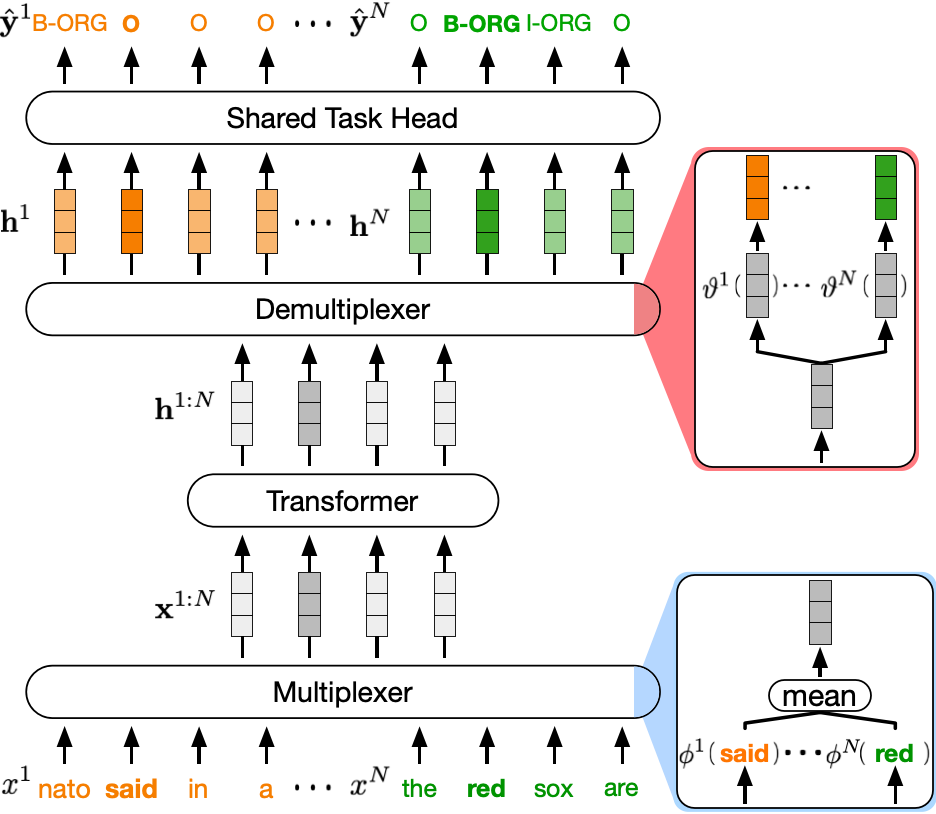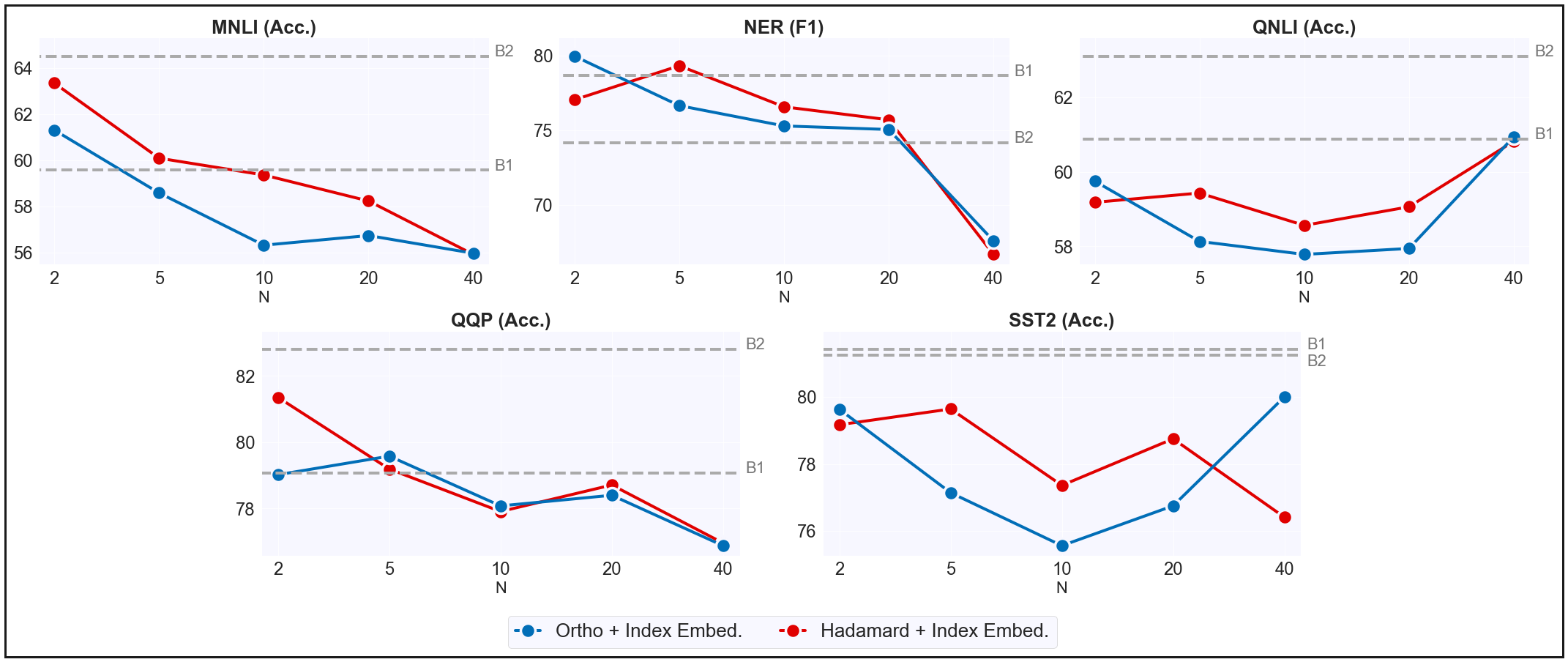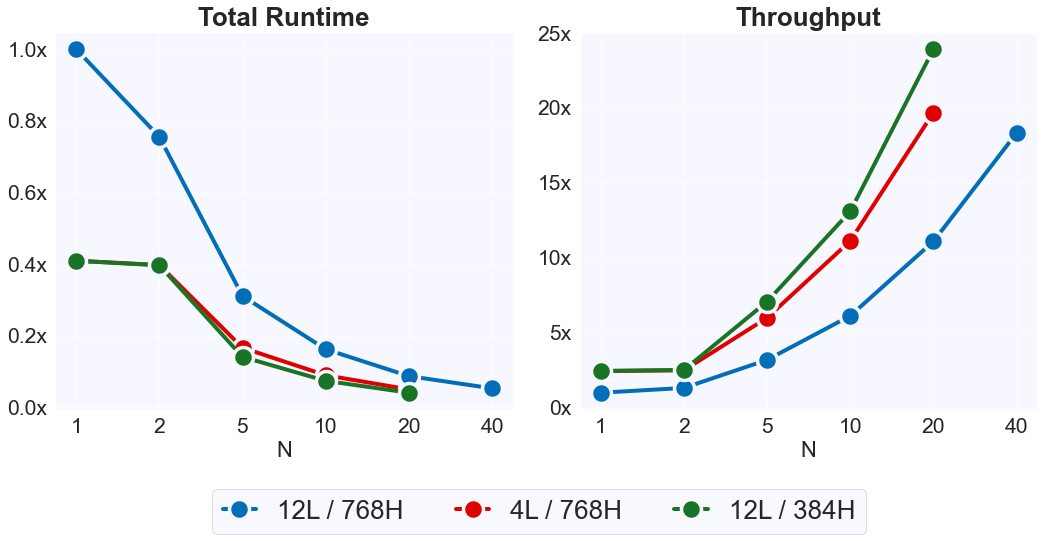## Abstract

In this work, we show that deep neural networks are capable of generating accurate predictions over mixtures of inputs. We introduce data multiplexing (DataMUX), a novel technique that enables networks to process multiple inputs simultaneously using a single compact representation, resulting in increased throughput with minimal extra space requirements. Our approach uses two key components – a multiplexing layer that performs a fixed linear transformation to each input before combining them to create a single mixed’ representation which is processed by the base network, and a demultiplexing layer that converts the network’s output back into independent representations before producing predictions for each input. We demonstrate the viability of DataMUX for multiple architectures (Transformers, and to a lesser extent MLPs and CNNs) across six different tasks spanning sentence classification, named entity recognition and image classification. For instance, DataMUX for Transformers can multiplex up to 20x/40x, achieving 11x/18x throughput with minimal absolute performance drops of <2% and <4%, respectively over a standard Transformer on MNLI, a natural language inference task.We also provide a theoretical construction for multiplexing in self-attention networks and analyze the effect of various design elements in DataMUX.

## Multiplexing for the TransformerIllustration of DataMUX applied to a Transformer model. Given a tuple of $$N$$ sentences ($$x^1, x^2, \dots, x^N$$), each of length $$L$$, we first apply a multiplexing operation which performs a transformation $$\phi^i$$ on the embeddings of each sequence $$x^i$$, such that the same transformation is applied to every token in a sequence $$x^i$$. The multiplexing operation then aggregates the sequences by averaging over each position, generating a single combined sequence $$\mathbf{x}^{1:N}\in\mathbb{R}^{L\times d}$$, for embedding size $$d$$, which will be passed on to the central Transformer model. After processing, we perform a demultiplexing operation to the Transformer model’s output $$\mathbf{h}^{1:N}\in\mathbb{R}^{L\times d}$$, to generate hidden representations $$\mathbf{h}^1, \mathbf{h}^2, \dots, \mathbf{h}^N$$, corresponding to inputs $$x^1, x^2, x^N$$ respectively. We finally use these hidden representations to generate predictions for a particular task (e.g. named entity recognition (NER)) using a shared task prediction head.Results for data multiplexing using $$\phi^i$$ as an orthogonal matrix or hadamard product. We compare 12-layer DataMUX models to a vanilla 12-layer Transformer (B1) and a Transformer pre-trained using our proposed retrieval task (B2). We demonstrate multiplexing up to $$40$$ instances without substantial degradation in task performance.

## Throughput Performance ResultsWe evaluate the efficiency of DataMUX for models of different sizes on $$20,000$$ MNLI instances ($$N=1$$ being a vanilla Transformer). The y-axis shows performance normalized by the 12-layer vanilla Transformer model. We demonstrate an $$18$$x throughput increase for $$N=40$$ on the 12-layer model and a nearly $$25$$x throughput increase for smaller models with $$N=20$$.

## Authors

@misc{murahari2022datamux,
`